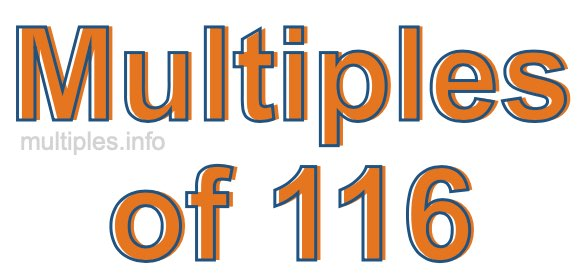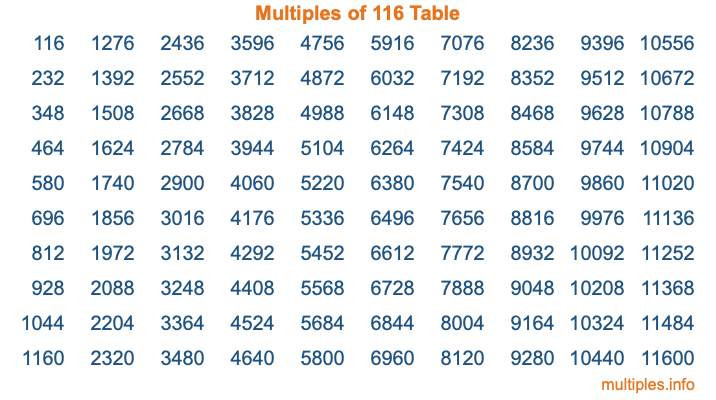Multiples of 116Welcome to the Multiples of 116 page. Here we will first teach you everything you will ever need to know about the multiples of 116, and then give you a study guide summary of everything we taught you to make sure you remember it all. Use this page to look up facts and learn information about the multiples of 116. This page will make you a multiples of one hundred sixteen expert!

Definition of Multiples of 116
Multiples of 116 are all the numbers that when divided by 116 equal an integer. Each of the multiples of 116 are called a multiple. A multiple of 116 is created by multiplying 116 by an integer.

Therefore, to create a list of multiples of 116, you start with 1 multiplied by 116, then 2 multiplied by 116, then 3 multiplied by 116, and so on for as long as you want. Thus, the list of the first five multiples of 116 is 116, 232, 348, 464, and 580. To see a larger list of multiples of 116, see the printable image of Multiples of 116 further down on this page. We also have a category where you can choose any nth multiple of 116.

Multiples of 116 Checker
The Multiples of 116 Checker below checks to see if any number of your choice is a multiple of 116. In other words, it checks to see if there is any number (integer) that when multiplied by 116 will equal your number. To do that, we divide your number by 116. If the the quotient is an integer, then your number is a multiple of 116.

Is  a multiple of 116?

Least Common Multiple of 116 and ...
A Least Common Multiple (LCM) is the lowest multiple that two or more numbers have in common. This is also called the smallest common multiple or lowest common multiple and is useful to know when you are adding our subtracting fractions. Enter one or more numbers below (116 is already entered) to find the LCM.

Check out our LCM Calculator if you need more details about the Least Common Multiple or if you need the LCM for different numbers for adding and subtraction fractions.

nth Multiple of 116
As we stated above, 116 is the first multiple of 116, 232 is the second multiple of 116, 348 is the third multiple of 116, and so on. Enter a number below to find the nth multiple of 116.

th multiple of 116

Multiples of 116 vs Factors of 116
116 is a multiple of 116 and a factor of 116, but that is where the similarities end. All postive multiples of 116 are 116 or greater than 116. All positive factors of 116 are 116 or less than 116.

Below is the beginning list of multiples of 116 and the factors of 116 so you can compare:

Multiples of 116: 116, 232, 348, 464, 580, etc.

Factors of 116: 1, 2, 4, 29, 58, 116

As you can see, the multiples of 116 are all the numbers that you can divide by 116 to get a whole number. The factors of 116, on the other hand, are all the whole numbers that you can multiply by another whole number to get 116.

It's also interesting to note that if a number (x) is a factor of 116, then 116 will also be a multiple of that number (x).

Multiples of 116 vs Divisors of 116
The divisors of 116 are all the integers that 116 can be divided by evenly. Below is a list of the divisors of 116.

Divisors of 116: 1, 2, 4, 29, 58, 116

The interesting thing to note here is that if you take any multiple of 116 and divide it by a divisor of 116, you will see that the quotient is an integer.

Multiples of 116 Table
Below is an image of the first 100 multiples of 116 in a table. The table is in chronological order, column by column. The first column has the first ten multiples of 116, the second column has the next ten multiples of 116, and so on.The Multiples of 116 Table is also referred to as the 116 Times Table or Times Table of 116. You are welcome to print out our table for your studies.

Negative Multiples of 116
Although not often discussed or needed in math, it is worth mentioning that you can make a list of negative multiples of 116 by multiplying 116 by -1, then by -2, then by -3, and so on, to get the following list of negative multiples of 116:

-116, -232, -348, -464, -580, etc.

Multiples of 116 Summary
Below is a summary of important Multiples of 116 facts that we have discussed on this page. To retain the knowledge on this page, we recommend that you read through the summary and explain to yourself or a study partner why they hold true.

There are an infinite number of multiples of 116.

A multiple of 116 divided by 116 will equal a whole number.

116 divided by a factor of 116 equals a divisor of 116.

The nth multiple of 116 is n times 116.

The largest factor of 116 is equal to the first positive multiple of 116.

116 is a multiple of every factor of 116.

116 is a multiple of 116.

A multiple of 116 divided by a divisor of 116 equals an integer.

116 divided by a divisor of 116 equals a factor of 116.

Any integer times 116 will equal a multiple of 116.

Multiples of a Number
Here you can get the multiples of another number, all with the same attention to detail as we did for multiples of 116 on this page.

Multiples of
Multiples of 117
Did you find our page about multiples of one hundred sixteen educational? Do you want more knowledge? Check out the multiples of the next number on our list!